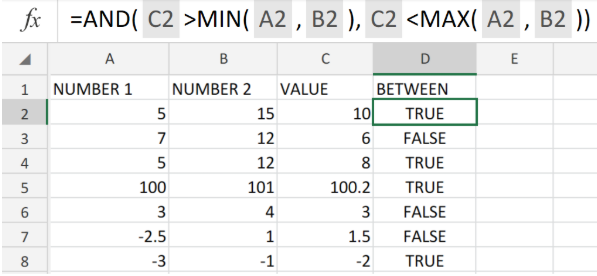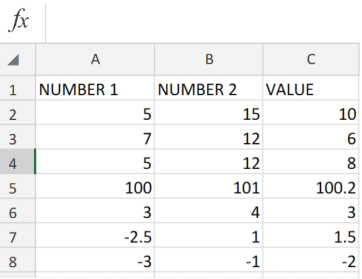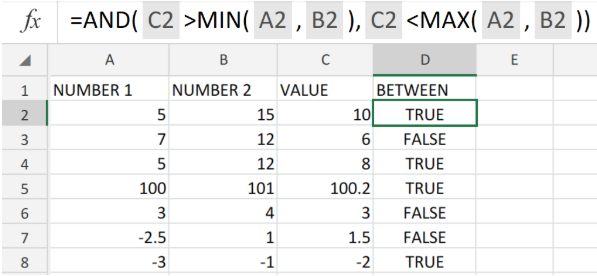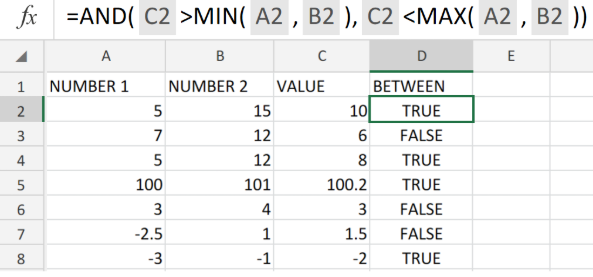Get instant live expert help with Excel or Google Sheets“My Excelchat expert helped me in less than 20 minutes, saving me what would have been 5 hours of work!”

#### Post your problem and you’ll get Expert help in seconds.

Your message must be at least 40 characters
Our professional Expert are available now. Your privacy is guaranteed.

# Value is between two numbersFigure 1. of the And Function in Excel.

Let’s say we have to determine whether or not a number value can be found between two numbers, we can utilize the Excel AND Function. This tutorial will walk through the process

## Generic Formula

`=AND(val>MIN(num1,num2),val<MAX(num1,num2))`

## How to use the AND, MIN AND MAX Functions in Excel

Basically, this formula syntax will run two tests on a value. We can achieve this by following two simple steps.

1. We will input our values/data on our Excel worksheet, including the values we are required to search for  as shown in Cells C2:C8 (column D) of the example illustrated below;Figure 2. of How to determine if a specific value can be found within a set of data values in Excel.

1. Label the next column in our worksheet as BETWEEN. This is where Excel will automatically generate the results of our search. Then proceed to enter the following formula syntax into the formula bar for cell D2 of our worksheet.

`=AND(C2>MIN(A2,B2),C2<MAX(A2,B2))`Figure 3. of the AND, MIN and MAX Functions in Excel.

Copy the formula syntax in D2 down into the formula bar for all the cells in the BETWEEN column to achieve the required results for our search.

We can also use the AND, MIN and MAX Function for situations where we have bulky data to statistically analyze. Our analysis involves that we find out whether a specific value can be found within the different sections of data that we have. We can conveniently utilize the “AND, MIN and MAX Functions for this.Figure 4. of Final Result.

## Instant Connection to an Expert through our Excelchat Service

Our live Excelchat Service is here for you. We have Excel Experts available 24/7 to answer any Excel questions you may have. Guaranteed connection within 30 seconds and a customized solution for you within 20 minutes.

### Did this post not answer your question? Get a solution from connecting with the expert.Another blog reader asked this question today on Excelchat:
Related blogs
Solution examplesI have a list of cost prices in one column and i would like to know what formula to use to add a percentage value. E.g if my cost price is just Â£1 i would like to create the sell price to be perhaps Â£3, but if my cost price is Â£1000, i would like the sell price to perhaps be Â£1,100 (not Â£3,000).
Solved by M. Y. in 60 minsHi, i need one formula, for example in salary level 200000 to 300000 is coming under 6B 300000 to 450000 is coming under 6A 450000 to 600000 is coming under 5A Now if salary is 280000 so which formula i can use to find their Band lavel
Solved by E. F. in 35 minswondering if there is a formula that when the name in one cell changes, the formula in the other cell changes? for example: working on a calculating cost for harvesting grain crops based on acres, bushels harvested, and bushels harvested over x/bushels per acre. in cell B3 i want when cell A1 says "wheat harvest" it will subtract 20 from cell B2, but when cell A1 says "corn harvest" i want to subtract 40 from cell B2 is this possible? thanks
Solved by T. D. in 20 minsHelp, I need a formula to measure if date entered in column e, is between date in column a and date in column b. please
Solved by V. U. in 20 minsSolved by G. U. in 19 mins## Subscribe to Excelchat.coAnother blog reader asked this question today on Excelchat: